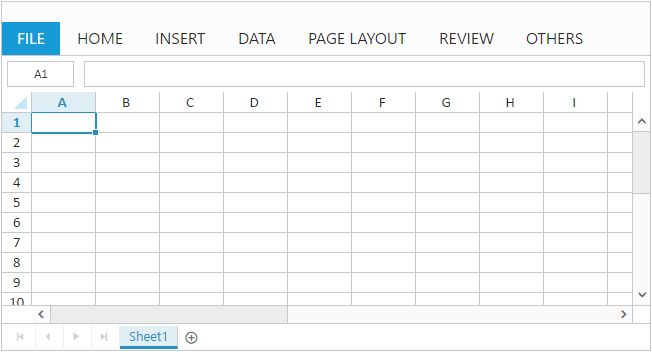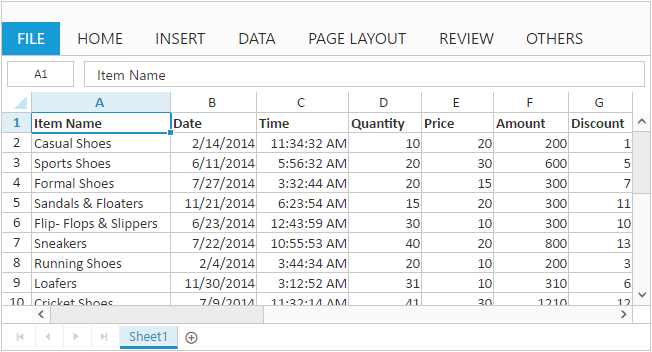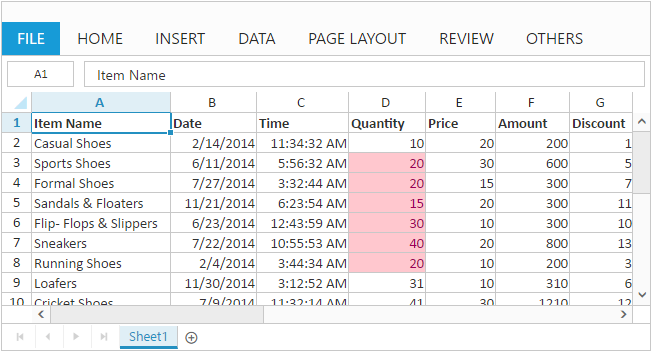# Getting Started

15 Mar 20199 minutes to read

This section explains briefly about how to create Spreadsheet in your ASP.NET MVC application and also how to populate the Spreadsheet with data, formats and how to export Spreadsheet data as excel file.

The following steps explains how to create spreadsheet,

1. Create Syncfusion ASP.NET MVC application. You can refer MVC Getting started documentation to create new project and add necessary dll’s and script files.
2. Add the following code example in view page to render Spreadsheet control. Spreadsheet is rendered with default height and width. You can also customize Spreadsheet dimension by setting `Height` and `Width` property in `ScrollSettings`.
``@(Html.EJ().Spreadsheet<object>("Spreadsheet"))``

Now, the Spreadsheet is rendered with default row and column count.Now, this section explains how to populate JSON data to the Spreadsheet. You can set `DataSource` property in `Sheets` settings to populate JSON data in Spreadsheet.

1) Create datasource for Spreadsheet control.

``````public ActionResult Index()
{
var DataSource = GetAllItemDetails().ToList();
ViewBag.Datasource = DataSource;
return View();
}

public IEnumerable<object> GetAllItemDetails()
{
List<ItemDetail> lItems = new List<ItemDetail>();
lItems.Add(new ItemDetail() { ItemName = "Casual Shoes", Date = new DateTime(2014, 02, 14), Time = new DateTime(2014, 02, 14, 11, 34, 32), Quantity = 10, Price = 20, Amount = 200, Discount = 1, Profit = 10 });
lItems.Add(new ItemDetail() { ItemName = "Sports Shoes", Date = new DateTime(2014, 06, 11), Time = new DateTime(2014, 06, 11, 05, 56, 32), Quantity = 20, Price = 30, Amount = 600, Discount = 5, Profit = 50 });
lItems.Add(new ItemDetail() { ItemName = "Formal Shoes", Date = new DateTime(2014, 07, 27), Time = new DateTime(2014, 07, 27, 03, 32, 44), Quantity = 20, Price = 15, Amount = 300, Discount = 7, Profit = 27 });
lItems.Add(new ItemDetail() { ItemName = "Sandals & Floaters", Date = new DateTime(2014, 11, 21), Time = new DateTime(2014, 11, 21, 06, 23, 54), Quantity = 15, Price = 20, Amount = 300, Discount = 11, Profit = 67 });
lItems.Add(new ItemDetail() { ItemName = "Flip- Flops & Slippers", Date = new DateTime(2014, 06, 23), Time = new DateTime(2014, 06, 23, 12, 43, 59), Quantity = 30, Price = 10, Amount = 300, Discount = 10, Profit = 70 });
lItems.Add(new ItemDetail() { ItemName = "Sneakers", Date = new DateTime(2014, 07, 22), Time = new DateTime(2014, 07, 22, 10, 55, 53), Quantity = 40, Price = 20, Amount = 800, Discount = 13, Profit = 66 });
lItems.Add(new ItemDetail() { ItemName = "Running Shoes", Date = new DateTime(2014, 02, 04), Time = new DateTime(2014, 02, 04, 03, 44, 34), Quantity = 20, Price = 10, Amount = 200, Discount = 3, Profit = 14 });
lItems.Add(new ItemDetail() { ItemName = "Loafers", Date = new DateTime(2014, 11, 30), Time = new DateTime(2014, 11, 30, 03, 12, 52), Quantity = 31, Price = 10, Amount = 310, Discount = 6, Profit = 29 });
lItems.Add(new ItemDetail() { ItemName = "Cricket Shoes", Date = new DateTime(2014, 07, 09), Time = new DateTime(2014, 07, 09, 11, 32, 14), Quantity = 41, Price = 30, Amount = 1210, Discount = 12, Profit = 166 });
lItems.Add(new ItemDetail() { ItemName = "T-Shirts", Date = new DateTime(2014, 10, 31), Time = new DateTime(2014, 10, 31, 12, 01, 44), Quantity = 50, Price = 10, Amount = 500, Discount = 9, Profit = 55 });
return lItems;
}

public class ItemDetail
{
public string ItemName { get; set; }
public DateTime Date { get; set; }
public DateTime Time { get; set; }
public int Quantity { get; set; }
public int Price { get; set; }
public int Amount { get; set; }
public int Discount { get; set; }
public int Profit { get; set; }
}``````

``````@(Html.EJ().Spreadsheet<object>("Spreadsheet")
.Sheets(sheet =>
{
sheet.RangeSettings(range =>
{
})
)``````## Apply Conditional formatting

Conditional formatting helps you to apply formats to a cell or range with certain color based on the cells values. You can use `AllowConditionalFormats` property to enable/disable Conditional formats.
To apply conditional formats for a range use `CFormatRule` property.The following code example illustrates this behavior,

``````@(Html.EJ().Spreadsheet<object>("Spreadsheet")
.Sheets(sheet =>
{
sheet.RangeSettings(range =>
{
}).CFormatRule(formatRule =>
{
})
)``````## Export Spreadsheet as Excel File

The Spreadsheet can save its data, style, format into an excel file. To enable save option in Spreadsheet set `AllowExporting` option in `ExportSettings` as `true`. Since Spreadsheet uses server side helper to save documents set `ExcelUrl` in `ExportSettings` option. The following code example illustrates this behavior,

``````@(Html.EJ().Spreadsheet<object>("Spreadsheet")
//...
.ExportSettings(export =>
{
export.ExcelUrl("ExportToExcel");
}
)
//...
)``````
``````[AcceptVerbs(HttpVerbs.Post)]
//...
public void ExportToExcel(string sheetModel, string sheetData, string password)
{
Use shortcut `Ctrl + S` to save Spreadsheet as excel file.
1. For more details about `Export` refer following `link`
2. For more details about `Client dependencies` refer the following `link`
3. For more details about `Server dependencies` refer the following `link`